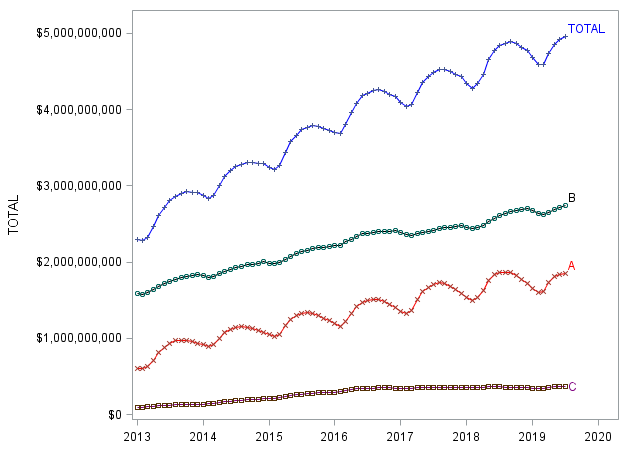## FORECAST month end balance using MULTIPLE VARIABLES

I am trying to forecast month end balances of the TOTAL portfolio using PROC ARIMA procedure. INside the portfolio there are 3 major product segments A, B and C. Each of which has a separate trend and seasonality as shown below in the graph

I wanted to try two methods,

method 1: Forecast of the TOTAL portfolio balance using PROC ARIMA (univariate)

method 2: Forecast of the TOTAL portfolio balance using PROC ARIMA (multi variate) using segment A, B and C BALANCES as input variables

Need help in forecasting the portoflio balance with multiple input variables, like average term , average interest rate, prepayments etc..

Which procedure can I use to achieve this?

Method 1 works fine but with method 2 I am getting these warnings

More values of input variable A are needed

More values of input variable B are needed

More values of input variable C are needed

METHOD 1:

proc arima data=upb_2 plots=all;
identify var=UPB(1,12) noprint;
estimate noconstant method=ml;
run;

METHOD 2:

proc arima data=UPB_INDUSTRY_2 plots=all;
identify var=TOTAL(1,12) noprint crosscorr=(A B C);
estimate input=(A B C)  noconstant method=ml;
run;1 ACCEPTED SOLUTION

Accepted Solutions

## Re: FORECAST month end balance using MULTIPLE VARIABLES

Hi @sivakoya ,

Method 2 in your example is an ARIMA model with input variables.  To forecast a response series using an ARIMA model with inputs, PROC ARIMA needs values of each of the input series for the LEAD= forecast horizon.  You can either provide future values of the input variables in the DATA= data set (setting the response variable to missing for those future observations) or you can use PROC ARIMA to model and forecast the input variables in addition to the response series.

For more details, please see the Forecasting with Input Variables section of the PROC ARIMA documentation at the following link:

https://go.documentation.sas.com/?docsetId=etsug&docsetTarget=etsug_arima_gettingstarted40.htm&docse...

I hope this helps!

DW

2 REPLIES 2

## Re: FORECAST month end balance using MULTIPLE VARIABLES

Hi @sivakoya ,

Method 2 in your example is an ARIMA model with input variables.  To forecast a response series using an ARIMA model with inputs, PROC ARIMA needs values of each of the input series for the LEAD= forecast horizon.  You can either provide future values of the input variables in the DATA= data set (setting the response variable to missing for those future observations) or you can use PROC ARIMA to model and forecast the input variables in addition to the response series.

For more details, please see the Forecasting with Input Variables section of the PROC ARIMA documentation at the following link:

https://go.documentation.sas.com/?docsetId=etsug&docsetTarget=etsug_arima_gettingstarted40.htm&docse...

I hope this helps!

DW

## Re: FORECAST month end balance using MULTIPLE VARIABLES

Thanks

Discussion stats
• 2 replies
• 1303 views
• 1 like
• 2 in conversation